# class TGraphPainter: public TVirtualGraphPainter

```
```

## The graph painter class

TGraphPainter paints TGraph, TGraphAsymmErrors, TGraphBentErrors, TGraphErrors and TGraphPolar.

### TGraph options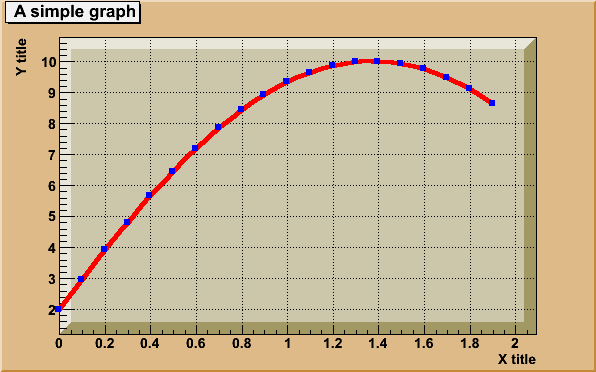```{
TCanvas *c1 = new TCanvas("c1","c1",200,10,600,400);

c1->SetFillColor(42);
c1->SetGrid();

const Int_t n = 20;
Double_t x[n], y[n];
for (Int_t i=0;i<n;i++) {
x[i] = i*0.1;
y[i] = 10*sin(x[i]+0.2);
}
gr = new TGraph(n,x,y);
gr->SetLineColor(2);
gr->SetLineWidth(4);
gr->SetMarkerColor(4);
gr->SetMarkerStyle(21);
gr->SetTitle("A simple graph");
gr->GetXaxis()->SetTitle("X title");
gr->GetYaxis()->SetTitle("Y title");
gr->Draw("ACP");

// TCanvas::Update() draws the frame, after which one can change it
c1->Update();
c1->GetFrame()->SetFillColor(21);
c1->GetFrame()->SetBorderSize(12);
c1->Modified();
return c1;
}```

### Exclusion graphs

When a graph is painted with the option "C" or "L" it is possible to draw a filled area on one side of the line. This is useful to show exclusion zones.

This drawing mode is activated when the absolute value of the graph line width (set thanks to SetLineWidth()) is greater than 99. In that case the line width number is interpreted as:

```      100*ff+ll = ffll
```
• The two digits number "ll" represent the normal line width
• The two digits number "ff" is the filled area width.
• The sign of "ffll" allows to flip the filled area from one side of the line to the other.
The current fill area attributes are used to draw the hatched zone.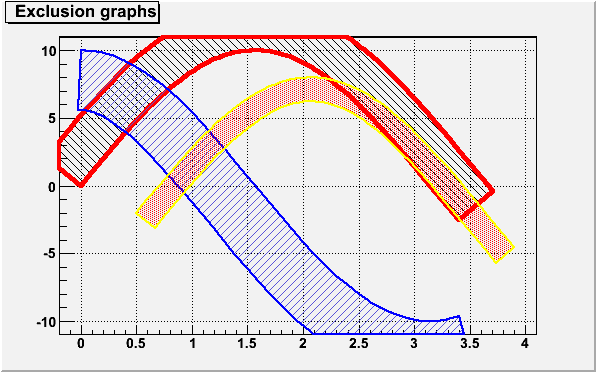```TCanvas *exclusiongraph() {
// Draw three graphs with an exclusion zone.
//Author: Olivier Couet

TCanvas *c1 = new TCanvas("c1","Exclusion graphs examples",200,10,600,400);
c1->SetGrid();

TMultiGraph *mg = new TMultiGraph();
mg->SetTitle("Exclusion graphs");

const Int_t n = 35;
Double_t x1[n], x2[n], x3[n], y1[n], y2[n], y3[n];
for (Int_t i=0;i<n;i++) {
x1[i]  = i*0.1;
x2[i]  = x1[i];
x3[i]  = x1[i]+.5;
y1[i] = 10*sin(x1[i]);
y2[i] = 10*cos(x1[i]);
y3[i] = 10*sin(x1[i])-2;
}

TGraph *gr1 = new TGraph(n,x1,y1);
gr1->SetLineColor(2);
gr1->SetLineWidth(1504);
gr1->SetFillStyle(3005);

TGraph *gr2 = new TGraph(n,x2,y2);
gr2->SetLineColor(4);
gr2->SetLineWidth(-2002);
gr2->SetFillStyle(3004);
gr2->SetFillColor(9);

TGraph *gr3 = new TGraph(n,x3,y3);
gr3->SetLineColor(5);
gr3->SetLineWidth(-802);
gr3->SetFillStyle(3002);
gr3->SetFillColor(2);

mg->Draw("AC");

return c1;
}```

### TGraphAsymmErrors options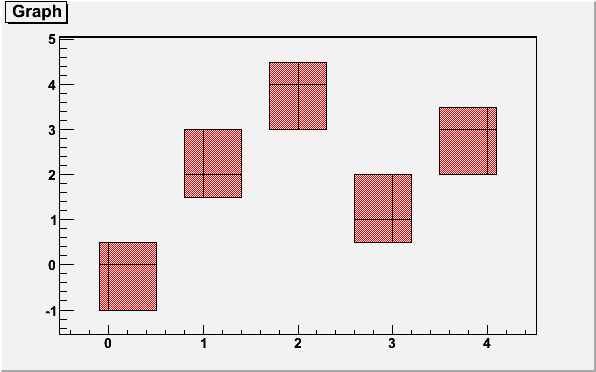```{
TCanvas *c2 = new TCanvas("c2","c2",200,10,600,400);
double ax[] = {0, 1, 2, 3, 4};
double ay[] = {0, 2, 4, 1, 3};
double aexl[] = {0.1, 0.2, 0.3, 0.4, 0.5};
double aexh[] = {0.5, 0.4, 0.3, 0.2, 0.1};
double aeyl[] = {1, 0.5, 1, 0.5, 1};
double aeyh[] = {0.5, 1, 0.5, 1, 0.5};
TGraphAsymmErrors* gae = new TGraphAsymmErrors(5, ax, ay, aexl, aexh, aeyl, aeyh);
gae->SetFillColor(2);
gae->SetFillStyle(3001);
gae->Draw("a2");
gae->Draw("p");
return c2;
}```

### TGraphBentErrors options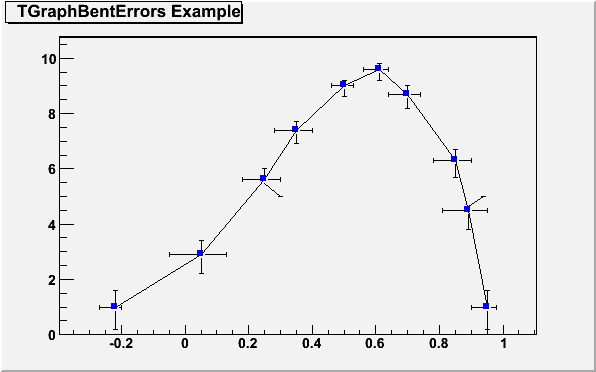```{
TCanvas *c3 = new TCanvas("c3","c3",200,10,600,400);
const Int_t n = 10;
Double_t x[n]  = {-0.22, 0.05, 0.25, 0.35, 0.5, 0.61,0.7,0.85,0.89,0.95};
Double_t y[n]  = {1,2.9,5.6,7.4,9,9.6,8.7,6.3,4.5,1};
Double_t exl[n] = {.05,.1,.07,.07,.04,.05,.06,.07,.08,.05};
Double_t eyl[n] = {.8,.7,.6,.5,.4,.4,.5,.6,.7,.8};
Double_t exh[n] = {.02,.08,.05,.05,.03,.03,.04,.05,.06,.03};
Double_t eyh[n] = {.6,.5,.4,.3,.2,.2,.3,.4,.5,.6};
Double_t exld[n] = {.0,.0,.0,.0,.0,.0,.0,.0,.0,.0};
Double_t eyld[n] = {.0,.0,.05,.0,.0,.0,.0,.0,.0,.0};
Double_t exhd[n] = {.0,.0,.0,.0,.0,.0,.0,.0,.0,.0};
Double_t eyhd[n] = {.0,.0,.0,.0,.0,.0,.0,.0,.05,.0};
TGraphBentErrors *gr = new TGraphBentErrors(n,x,y,exl,exh,eyl,eyh,exld,exhd,eyld,eyhd);
gr->SetTitle("TGraphBentErrors Example");
gr->SetMarkerColor(4);
gr->SetMarkerStyle(21);
gr->Draw("ALP");
return c3;
}```

### TGraphPolar options

The drawing options for the polar graphs are the following values:
"O" Polar labels are paint orthogonally to the polargram radius. Polymarker are paint at each point position. Paint error bars. Paint fill area (closed polygon). Force axis redrawing even if a polargram already exists. Disable the display of the polar labels.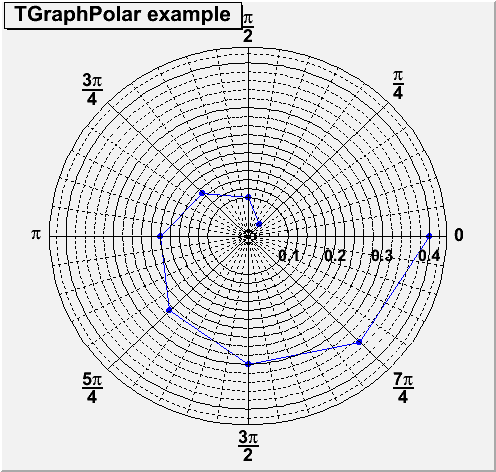```{
TCanvas *c1 = new TCanvas("c1","c1",500,500);
TGraphPolar * grP1 = new TGraphPolar();
grP1->SetTitle("TGraphPolar example");

grP1->SetPoint(0, (1*TMath::Pi())/4., 0.05);
grP1->SetPoint(1, (2*TMath::Pi())/4., 0.10);
grP1->SetPoint(2, (3*TMath::Pi())/4., 0.15);
grP1->SetPoint(3, (4*TMath::Pi())/4., 0.20);
grP1->SetPoint(4, (5*TMath::Pi())/4., 0.25);
grP1->SetPoint(5, (6*TMath::Pi())/4., 0.30);
grP1->SetPoint(6, (7*TMath::Pi())/4., 0.35);
grP1->SetPoint(7, (8*TMath::Pi())/4., 0.40);

grP1->SetMarkerStyle(20);
grP1->SetMarkerSize(1.);
grP1->SetMarkerColor(4);
grP1->SetLineColor(4);
grP1->Draw("ALP");

// Update, otherwise GetPolargram returns 0
c1->Update();

return c1;
}```
```
```

## Function Members (Methods)

public:
 TGraphPainter() TGraphPainter(const TGraphPainter&) virtual ~TGraphPainter() void TObject::AbstractMethod(const char* method) const virtual void TObject::AppendPad(Option_t* option = "") virtual void TObject::Browse(TBrowser* b) static TClass* Class() virtual const char* TObject::ClassName() const virtual void TObject::Clear(Option_t* = "") virtual TObject* TObject::Clone(const char* newname = "") const virtual Int_t TObject::Compare(const TObject* obj) const void ComputeLogs(Int_t npoints, Int_t opt) virtual void TObject::Copy(TObject& object) const virtual void TObject::Delete(Option_t* option = "")MENU virtual Int_t TObject::DistancetoPrimitive(Int_t px, Int_t py) virtual Int_t DistancetoPrimitiveHelper(TGraph* theGraph, Int_t px, Int_t py) virtual void TObject::Draw(Option_t* option = "") virtual void TObject::DrawClass() constMENU virtual TObject* TObject::DrawClone(Option_t* option = "") constMENU virtual void DrawPanelHelper(TGraph* theGraph) virtual void TObject::Dump() constMENU virtual void TObject::Error(const char* method, const char* msgfmt) const virtual void TObject::Execute(const char* method, const char* params, Int_t* error = 0) virtual void TObject::Execute(TMethod* method, TObjArray* params, Int_t* error = 0) virtual void TObject::ExecuteEvent(Int_t event, Int_t px, Int_t py) virtual void ExecuteEventHelper(TGraph* theGraph, Int_t event, Int_t px, Int_t py) virtual void TObject::Fatal(const char* method, const char* msgfmt) const virtual TObject* TObject::FindObject(const char* name) const virtual TObject* TObject::FindObject(const TObject* obj) const virtual Option_t* TObject::GetDrawOption() const static Long_t TObject::GetDtorOnly() virtual const char* TObject::GetIconName() const virtual const char* TObject::GetName() const virtual char* TObject::GetObjectInfo(Int_t px, Int_t py) const virtual char* GetObjectInfoHelper(TGraph* theGraph, Int_t px, Int_t py) const static Bool_t TObject::GetObjectStat() virtual Option_t* TObject::GetOption() const static TVirtualGraphPainter* TVirtualGraphPainter::GetPainter() virtual const char* TObject::GetTitle() const virtual UInt_t TObject::GetUniqueID() const virtual Bool_t TObject::HandleTimer(TTimer* timer) virtual ULong_t TObject::Hash() const virtual void TObject::Info(const char* method, const char* msgfmt) const virtual Bool_t TObject::InheritsFrom(const char* classname) const virtual Bool_t TObject::InheritsFrom(const TClass* cl) const virtual void TObject::Inspect() constMENU void TObject::InvertBit(UInt_t f) virtual TClass* IsA() const virtual Bool_t TObject::IsEqual(const TObject* obj) const virtual Bool_t TObject::IsFolder() const Bool_t TObject::IsOnHeap() const virtual Bool_t TObject::IsSortable() const Bool_t TObject::IsZombie() const virtual void TObject::ls(Option_t* option = "") const void TObject::MayNotUse(const char* method) const virtual Bool_t TObject::Notify() static void TObject::operator delete(void* ptr) static void TObject::operator delete(void* ptr, void* vp) static void TObject::operator delete[](void* ptr) static void TObject::operator delete[](void* ptr, void* vp) void* TObject::operator new(size_t sz) void* TObject::operator new(size_t sz, void* vp) void* TObject::operator new[](size_t sz) void* TObject::operator new[](size_t sz, void* vp) TGraphPainter& operator=(const TGraphPainter&) virtual void TObject::Paint(Option_t* option = "") virtual void PaintGraph(TGraph* theGraph, Int_t npoints, const Double_t* x, const Double_t* y, Option_t* chopt) void PaintGraphAsymmErrors(TGraph* theGraph, Option_t* option) void PaintGraphBentErrors(TGraph* theGraph, Option_t* option) void PaintGraphErrors(TGraph* theGraph, Option_t* option) virtual void PaintGrapHist(TGraph* theGraph, Int_t npoints, const Double_t* x, const Double_t* y, Option_t* chopt) void PaintGraphPolar(TGraph* theGraph, Option_t* option) void PaintGraphQQ(TGraph* theGraph, Option_t* option) void PaintGraphSimple(TGraph* theGraph, Option_t* option) virtual void PaintHelper(TGraph* theGraph, Option_t* option) void PaintPolyLineHatches(TGraph* theGraph, Int_t n, const Double_t* x, const Double_t* y) virtual void PaintStats(TGraph* theGraph, TF1* fit) virtual void TObject::Pop() virtual void TObject::Print(Option_t* option = "") const virtual Int_t TObject::Read(const char* name) virtual void TObject::RecursiveRemove(TObject* obj) void TObject::ResetBit(UInt_t f) virtual void TObject::SaveAs(const char* filename = "", Option_t* option = "") constMENU virtual void TObject::SavePrimitive(basic_ostream >& out, Option_t* option = "") void TObject::SetBit(UInt_t f) void TObject::SetBit(UInt_t f, Bool_t set) virtual void TObject::SetDrawOption(Option_t* option = "")MENU static void TObject::SetDtorOnly(void* obj) static void TObject::SetObjectStat(Bool_t stat) static void TVirtualGraphPainter::SetPainter(TVirtualGraphPainter* painter) virtual void TObject::SetUniqueID(UInt_t uid) virtual void ShowMembers(TMemberInspector& insp, char* parent) void Smooth(TGraph* theGraph, Int_t npoints, Double_t* x, Double_t* y, Int_t drawtype) virtual void Streamer(TBuffer& b) void StreamerNVirtual(TBuffer& b) virtual void TObject::SysError(const char* method, const char* msgfmt) const Bool_t TObject::TestBit(UInt_t f) const Int_t TObject::TestBits(UInt_t f) const virtual void TObject::UseCurrentStyle() virtual void TObject::Warning(const char* method, const char* msgfmt) const virtual Int_t TObject::Write(const char* name = 0, Int_t option = 0, Int_t bufsize = 0) virtual Int_t TObject::Write(const char* name = 0, Int_t option = 0, Int_t bufsize = 0) const
protected:
 virtual void TObject::DoError(int level, const char* location, const char* fmt, va_list va) const void TObject::MakeZombie()

## Data Members

public:
 enum TObject::EStatusBits { kCanDelete kMustCleanup kObjInCanvas kIsReferenced kHasUUID kCannotPick kNoContextMenu kInvalidObject }; enum TObject::[unnamed] { kIsOnHeap kNotDeleted kZombie kBitMask kSingleKey kOverwrite kWriteDelete };

## Class Charts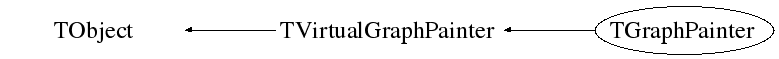## Function documentation

Default constructor

```
```

Destructor.

```
```
void ComputeLogs(Int_t npoints, Int_t opt)
Compute the lorarithm of global variables gxwork and gywork
according to the value of Options and put the results in the global
variables gxworkl and gyworkl.

npoints : Number of points in gxwork and in gywork.

• opt = 1 CompulteLogs is called from PaintGrapHist
• opt = 0 CompulteLogs is called from PaintGraph
```
```
Int_t DistancetoPrimitiveHelper(TGraph* theGraph, Int_t px, Int_t py)
Compute distance from point px,py to a graph.

Compute the closest distance of approach from point px,py to this line. The distance is computed in pixels units.

```
```
void DrawPanelHelper(TGraph* theGraph)
Display a panel with all histogram drawing options.

```
```
void ExecuteEventHelper(TGraph* theGraph, Int_t event, Int_t px, Int_t py)
Execute action corresponding to one event.

This member function is called when a graph is clicked with the locator.

If the left mouse button is clicked on one of the line end points, this point follows the cursor until button is released.

If the middle mouse button clicked, the line is moved parallel to itself until the button is released.

```
```
char * GetObjectInfoHelper(TGraph* theGraph, Int_t px, Int_t py) const
void PaintHelper(TGraph* theGraph, Option_t* option)
``` Paint a any kind of TGraph
```
void PaintGraph(TGraph* theGraph, Int_t npoints, const Double_t* x, const Double_t* y, Option_t* chopt)
``` Control function to draw a graph.

Draws one dimensional graphs. The aspect of the graph is done
according to the value of the chopt.

Input parameters:

npoints : Number of points in X or in Y.
x[npoints] or x : X coordinates or (XMIN,XMAX) (WC space).
y[npoints] or y : Y coordinates or (YMIN,YMAX) (WC space).
chopt : Option.

chopt='L' :  A simple polyline between every points is drawn

chopt='F' :  A fill area is drawn ('CF' draw a smooth fill area)

chopt='A' :  Axis are drawn around the graph

chopt='C' :  A smooth Curve is drawn

chopt='*' :  A Star is plotted at each point

chopt='P' :  Idem with the current marker

chopt='B' :  A Bar chart is drawn at each point

chopt='1' :  ylow=rwymin

chopt='X+' : The X-axis is drawn on the top side of the plot.

chopt='Y+' : The Y-axis is drawn on the right side of the plot.

When a graph is painted with the option "C" or "L" it is possible to draw
a filled area on one side of the line. This is useful to show exclusion
zones. This drawing mode is activated when the absolute value of the
graph line width (set thanks to SetLineWidth) is greater than 99. In that
case the line width number is interpreted as 100*ff+ll = ffll . The two
digits number "ll" represent the normal line width whereas "ff" is the
filled area width. The sign of "ffll" allows to flip the filled area
from one side of the line to the other. The current fill area attributes
are used to draw the hatched zone.
```
void PaintGrapHist(TGraph* theGraph, Int_t npoints, const Double_t* x, const Double_t* y, Option_t* chopt)
``` Control function to draw a graphistogram.

Draws one dimensional graphs. The aspect of the graph is done
according to the value of the chopt.

Input parameters:

npoints : Number of points in X or in Y.
X(N) or x : X coordinates or (XMIN,XMAX) (WC space).
Y(N) or y : Y coordinates or (YMIN,YMAX) (WC space).
chopt : Option.

chopt='R' :  Graph is drawn horizontaly, parallel to X axis.
(default is vertically, parallel to Y axis)
If option R is selected the user must give:
2 values for Y (y=YMIN and y=YMAX)
N values for X, one for each channel.
Otherwise the user must give:
N values for Y, one for each channel.
2 values for X (x=XMIN and x=XMAX)

chopt='L' :  A simple polyline beetwen every points is drawn

chopt='H' :  An Histogram with equidistant bins is drawn
as a polyline.

chopt='F' :  An histogram with equidistant bins is drawn
as a fill area. Contour is not drawn unless
chopt='H' is also selected..

chopt='N' :  Non equidistant bins (default is equidistant)
If N is the number of channels array X and Y
must be dimensionned as follow:
If option R is not selected (default) then
the user must give:
(N+1) values for X (limits of channels).
N values for Y, one for each channel.
Otherwise the user must give:
(N+1) values for Y (limits of channels).
N values for X, one for each channel.

chopt='F1':  Idem as 'F' except that fill area is no more
reparted arround axis X=0 or Y=0 .

chopt='F2':  Draw a Fill area polyline connecting the center of bins

chopt='C' :  A smooth Curve is drawn.

chopt='*' :  A Star is plotted at the center of each bin.

chopt='P' :  Idem with the current marker
chopt='P0':  Idem with the current marker. Empty bins also drawn

chopt='B' :  A Bar chart with equidistant bins is drawn as fill
areas (Contours are drawn).

chopt='9' :  Force graph to be drawn in high resolution mode.
By default, the graph is drawn in low resolution
in case the number of points is greater than the number of

chopt='][' : "Cutoff" style. When this option is selected together with
H option, the first and last vertical lines of the histogram
are not drawn.
```
void PaintGraphAsymmErrors(TGraph* theGraph, Option_t* option)
``` Paint this TGraphAsymmErrors with its current attributes.

By default horizonthal and vertical small lines are drawn at
the end of the error bars. if option "z" or "Z" is specified,
these lines are not drawn.

If option contains ">" an arrow is drawn at the end of the error bars
if option contains "|>" a full arrow is drawn at the end of the error bars
the size of the arrow is set to 2/3 of the marker size.

By default, error bars are drawn. If option "X" is specified,
the errors are not drawn (TGraph::Paint equivalent).

If option "||" is specified only the end vertical/horizonthal lines
of the error bars are drawn. This option is interesting to superimpose
systematic errors on top of a graph with statistical errors. The option
"[]" does the same except that it draws additionnal tick marks at the
end of the vertical/horizonthal lines. This makes less ambiguous plots
in case several graphs are drawn on the same picture.

If option "2" is specified error rectangles are drawn.

If option "3" is specified a filled area is drawn through the end points
of the vertical error bars.

If option "4" is specified a smoothed filled area is drawn through the end
points of the vertical error bars.
```
void PaintGraphBentErrors(TGraph* theGraph, Option_t* option)
``` Paint this TGraphBentErrors with its current attributes.

By default horizonthal and vertical small lines are drawn at
the end of the error bars. if option "z" or "Z" is specified,
these lines are not drawn.

If option contains ">" an arrow is drawn at the end of the error bars
if option contains "|>" a full arrow is drawn at the end of the error bars
the size of the arrow is set to 2/3 of the marker size.

By default, error bars are drawn. If option "X" is specified,
the errors are not drawn (TGraph::Paint equivalent).

If option "||" is specified only the end vertical/horizonthal lines
of the error bars are drawn. This option is interesting to superimpose
systematic errors on top of a graph with statistical errors. The option
"[]" does the same except that it draws additionnal tick marks at the
end of the vertical/horizonthal lines. This makes less ambiguous plots
in case several graphs are drawn on the same picture.

If option "3" is specified a filled area is drawn through the end points
of the vertical error bars.

If option "4" is specified a smoothed filled area is drawn through the end
points of the vertical error bars.
```
void PaintGraphErrors(TGraph* theGraph, Option_t* option)
``` Paint this TGraphErrors with its current attributes.

By default horizonthal and vertical small lines are drawn at
the end of the error bars. if option "z" or "Z" is specified,
these lines are not drawn.

If option contains ">" an arrow is drawn at the end of the error bars
if option contains "|>" a full arrow is drawn at the end of the error bars
the size of the arrow is set to 2/3 of the marker size.

By default, error bars are drawn. If option "X" is specified,
the errors are not drawn (TGraph::Paint equivalent).

If option "||" is specified only the end vertical/horizonthal lines
of the error bars are drawn. This option is interesting to superimpose
systematic errors on top of a graph with statistical errors. The option
"[]" does the same except that it draws additionnal tick marks at the
end of the vertical/horizonthal lines. This makes less ambiguous plots
in case several graphs are drawn on the same picture.

If option "2" is specified error rectangles are drawn.

If option "3" is specified a filled area is drawn through the end points
of the vertical error bars.

If option "4" is specified a smoothed filled area is drawn through the end
points of the vertical error bars.

Use gStyle->SetErrorX(dx) to control the size of the error along x.
set dx = 0 to suppress the error along x.

Use gStyle->SetEndErrorSize(np) to control the size of the lines
at the end of the error bars (when option 1 is used).
By default np=1. (np represents the number of pixels).
```
void PaintGraphPolar(TGraph* theGraph, Option_t* option)
``` Paint TGraphPolar.

"options" can have the following values:
- "O" Polar labels are paint orthogonally to the polargram radius.
- "P" Polymarker are paint at each point position.
- "E" Paint error bars.
- "F" Paint fill area (closed polygon).
- "A" Force axis redrawing even if a polargram already exists.
- "N" Disable the display of the polar labels.
```
void PaintGraphQQ(TGraph* theGraph, Option_t* option)
``` Paint this graphQQ. No options for the time being.
```
void PaintGraphSimple(TGraph* theGraph, Option_t* option)
``` Paint a simple graph, without errors bars.
```
void PaintPolyLineHatches(TGraph* theGraph, Int_t n, const Double_t* x, const Double_t* y)
Paint a polyline with hatches on one side showing an exclusion
zone. x and y are the the vectors holding the polyline and n the
number of points in the polyline and w the width of the hatches.
w can be negative.
This method is not meant to be used directly. It is called
automatically according to the line style convention.

```
```
void PaintStats(TGraph* theGraph, TF1* fit)
```  Paint "stats" box with the fit info.
```
void Smooth(TGraph* theGraph, Int_t npoints, Double_t* x, Double_t* y, Int_t drawtype)
``` Smooth a curve given by N points.

Underlaying routine for Draw based on the CERN GD3 routine TVIPTE

Author - Marlow etc.   Modified by - P. Ward     Date -  3.10.1973

This routine draws a smooth tangentially continuous curve through
the sequence of data points P(I) I=1,N where P(I)=(X(I),Y(I))
the curve is approximated by a polygonal arc of short vectors .
the data points can represent open curves, P(1) != P(N) or closed
curves P(2) == P(N) . If a tangential discontinuity at P(I) is
required , then set P(I)=P(I+1) . loops are also allowed .

Reference Marlow and Powell,Harwell report No.R.7092.1972
MCCONALOGUE,Computer Journal VOL.13,NO4,NOV1970Pp392 6

npoints   : Number of data points.
x         : Abscissa
y         : Ordinate
```

Author: Olivier Couet
Last change: root/histpainter:\$Id: TGraphPainter.h,v 1.00
Last generated: 2008-12-10 08:21